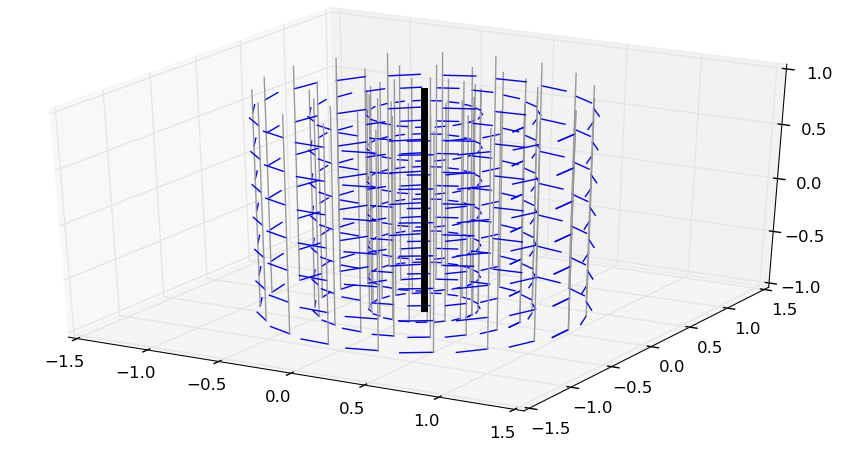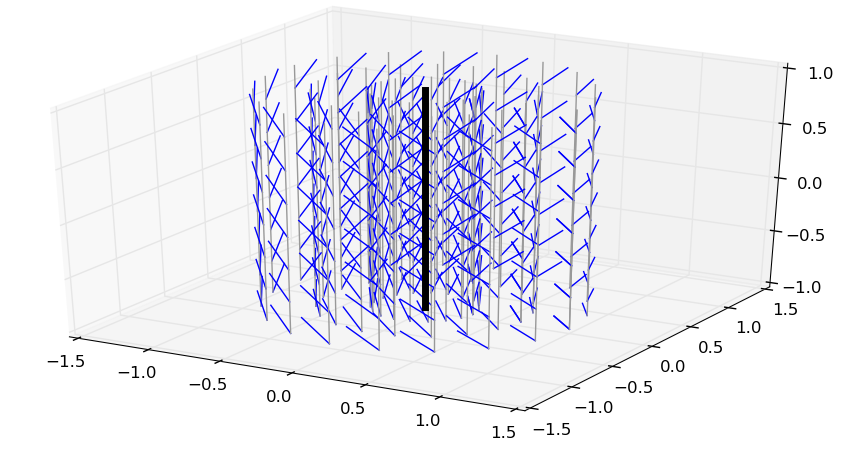# Screw axes

Screws, the mathematical objects used to describe rigid body motions and forces, are intrinsically linked with axes, be it axes of motion (such as the rotation axis of a pure rotation) or force (such as the line of action of a force).

## Central and noncentral axes

The central axis of a screw $$\hat\bfs$$ is the axis directed by the resultant $$\bfr$$ where the moment $$\bfm_P$$ is parallel to $$\bfr$$ (not necessarily zero). A noncentral axis of the screw is any other axis in space directed by the resultant $$\bfr$$. From Varignon's formula, any two points $$P$$ and $$Q$$ belonging to a noncentral axis have the same moment $$\bfm_P = \bfm_Q$$. This way, the moment field can be seen as a vector field over noncentral axes (5D space) rather than over points (6D space).

Another interesting value for a screw is its automoment, which is the scalar product $$(\bfr \cdot \bfm_P)$$ between its resultant and its moment. Again, from Varignon's formula, it does not depend on the point $$P \in \mathsf{E}^3$$ selected to compute it. In particular, on the central axis $$\Delta_c$$, the moment is given by:

\begin{equation} \bfm_{\Delta_c} \ = \ (\bfr \cdot \bfm_{\Delta_c})\,\frac{\bfr}{\| \bfr \|^2} \ = \ \rho(\hat\bfs)\,\bfr \end{equation}

where $$\rho(\hat\bfs) = (\bfr \cdot \bfm_P) / (\bfr \cdot \bfr)$$ is the pitch of the screw. A screw is fully defined by:

• its pitch $$\rho(\hat\bfs)$$,
• its central axis $$\Delta_c$$, and
• its magnitude $$\| \bfr \|$$.

Going back to Chasles' theorem on twists:

• the central axis $$\Delta_c$$ is the axis of the motion,
• the magnitude $$\| \bfomega \|$$ gives the amount of the rotation around $$\Delta_c$$, and
• the pitch $$\rho(\hat\bft) = (\bfomega \cdot \bfv_P) / (\bfomega \cdot \bfomega)$$ gives the amount of translation along $$\Delta_c$$.

## Illustration

Here is a small Python function with mplot3d to plot the moment field of a screw, assuming $$\Delta_c$$ goes through the origin:

from matplotlib.pyplot import figure, show, ion
from mpl_toolkits.mplot3d import Axes3D
from numpy import linspace, cos, sin, arange, pi, array, cross

def plot_screw(res, pitch, scale=0.2):
res = array(res)  # just in case
fig = figure()
ax = fig.gca(projection='3d')
for r in linspace(0., 1., 4):
for theta in linspace(0, 2 * pi, 20):
x, y = r * cos(theta), r * sin(theta)
ax.plot([x - res, x + res], [y - res, y + res],
[-1, 1], color='#999999')
for z in arange(-1., 1., 0.3):
u, v, w = scale * (cross([x, y, z], res) + pitch * res)
ax.plot([x, x + u], [y, y + v], [z, z + w], color='b')
ax.plot([-res, +res], [-res, +res], [-1, 1], color='k', lw=5)
show()


Here is the example of a screw with zero pitch, such as a pure rotation. The bold black line shows the central axis, while the thin gray lines are for noncentral ones. The moment field (linear velocities) is in blue.Now, the same with a pitch $$\rho(\hat\bft) = 1$$ m/rad, meaning each radian of rotation brings a one meter translation:We see where the word “screw” comes from: following the moment field of the screw above is like following the thread of an actual screw.

## To go further

An excellent read is the seminal 2004 survey paper by Sardain and Bessonnet: Forces Acting on a Biped Robot. Center of Pressure—Zero Moment Point. It uses of the axial representation of screws to gain insight on CoP and ZMP, two characteristic points for contact.

Pages of this website are under the CC-BY 4.0 license.